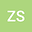•A symmetric bi additive mapping $D$, on a prime ring $R$ is called skew symmetric bi-Jordan derivation if it satisfies the following condition associated with the automorphism of $\alpha$ of $R$:% \begin{equation*} D( x^{2},z) =\alpha(x)D(x,z) +D(x,z)x \end{equation*}% The purpose of this paper is to prove some results concerning skew symmetric bi-Jordan derivation, as a generalization of symmetric bi-Jordan derivation.